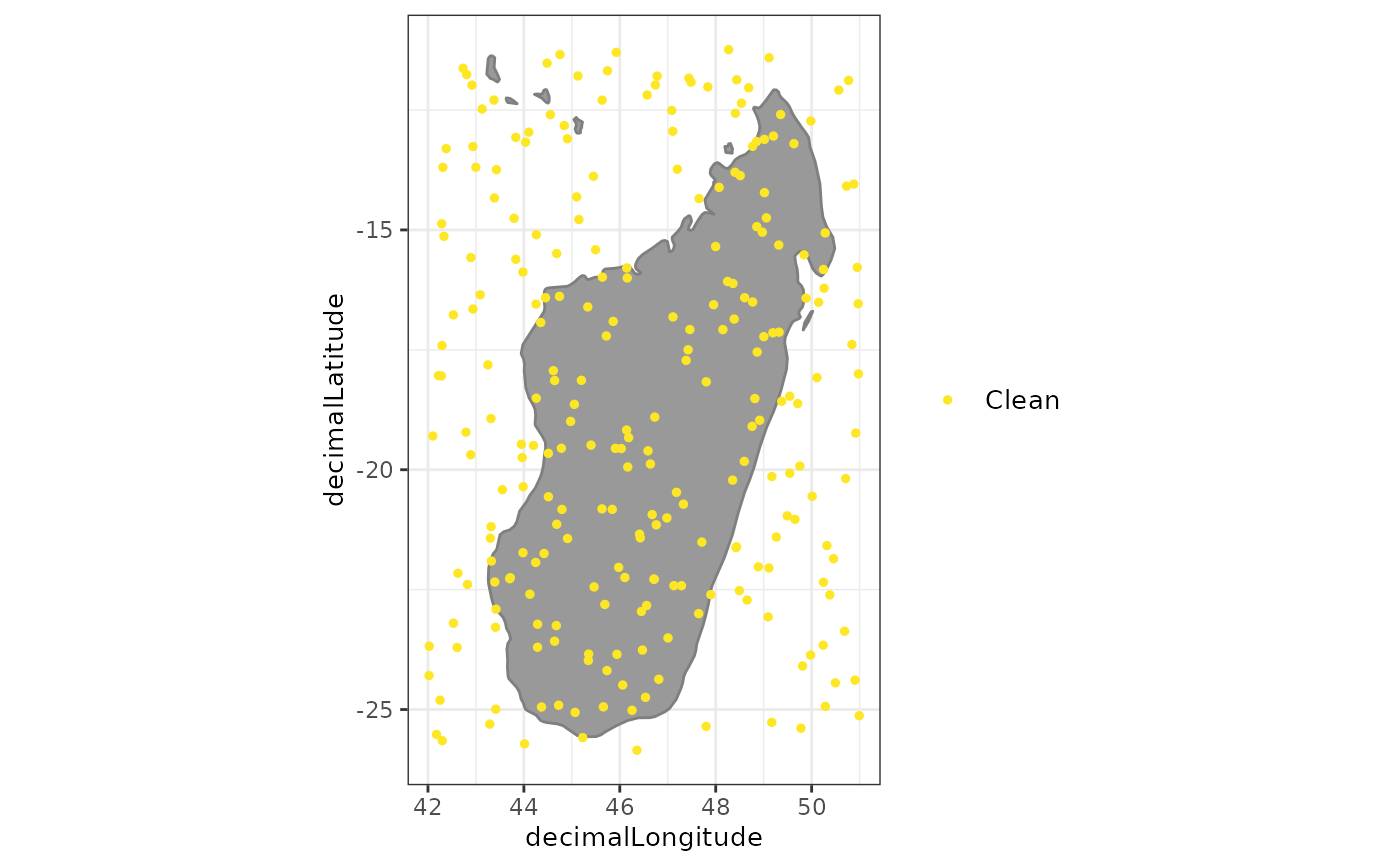A set of plots to explore objects of the class `spatialvalid`. A plot to visualize the flags from clean_coordinates

## Usage

``````# S3 method for spatialvalid
plot(
x,
lon = "decimalLongitude",
lat = "decimalLatitude",
bgmap = NULL,
clean = TRUE,
details = FALSE,
pts_size = 1,
font_size = 10,
zoom_f = 0.1,
...
)``````

## Arguments

x

an object of the class `spatialvalid` as from `clean_coordinates`.

lon

character string. The column with the longitude coordinates. Default = “decimalLongitude”.

lat

character string. The column with the latitude coordinates. Default = “decimalLatitude”.

bgmap

an object of the class `SpatVector` or `sf` used as background map. Default = ggplot::borders()

clean

logical. If TRUE, non-flagged coordinates are included in the map.

details

logical. If TRUE, occurrences are color-coded by the type of flag.

pts_size

numeric. The point size for the plot.

font_size

numeric. The font size for the legend and axes

zoom_f

numeric. the fraction by which to expand the plotting area from the occurrence records. Increase, if countries do not show up on the background map.

...

arguments to be passed to methods.

## Value

A plot of the records flagged as potentially erroneous by `clean_coordinates`.

`clean_coordinates`

## Examples

``````

exmpl <- data.frame(species = sample(letters, size = 250, replace = TRUE),
decimalLongitude = runif(250, min = 42, max = 51),
decimalLatitude = runif(250, min = -26, max = -11))

test <- clean_coordinates(exmpl, species = "species",
tests = c("sea", "gbif", "zeros"),
verbose = FALSE)

summary(test)
#>     .val     .zer     .gbf .summary
#>        0        0        0        0
plot(test)``````